# scikit-learn简介

scikit-learn，又写作sklearn，是一个开源的基于python语言的机器学习工具包。它通过NumPy, SciPy和
Matplotlib等python数值计算的库实现高效的算法应用，并且涵盖了几乎所有主流机器学习算法。
http://scikit-learn.org/stable/index.html
在工程应用中，用python手写代码来从头实现一个算法的可能性非常低，这样不仅耗时耗力，还不一定能够写出

sklearn有一个完整而丰富的官网，里面讲解了基于sklearn对所有算法的实现和简单应用。然而，这个官网是全英

sklearn中的实现，如果希望详细了解算法的原理，建议阅读下面这本两本书：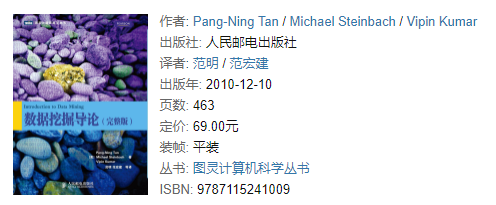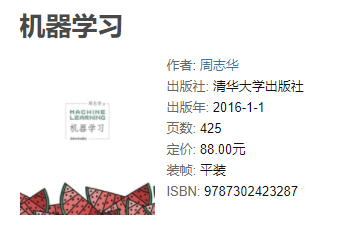# 决策树原理

1.1 决策树是如何工作# sklearn中的决策树

## 一、建立一棵树

### 1、导入需要的算法库和模块

``````#预定义
from sklearn import tree               #导入模型
from .datasets import load_wine        #加载红酒数据  共178个样本  代表了红酒的三个档次（分别有59,71,48个样本） 以及与之对应的13维的属性数据
from sklearn.model_selection import train_test_split#制作数据集和测试集``````

### 2. 探索数据

``````wine = load_wine()
wine.data.shape#形状 （178,13）
wine.target#（178，）
#如果wine是一张表，应该长这样：
import pandas as pd
pd.concat([pd.DataFrame(wine.data),pd.DataFrame(wine.target)],axis=1)
wine.feature_names
wine.target_names``````

### 3. 分训练集和测试集

``````Xtrain, Xtest, Ytrain, Ytest = train_test_split(wine.data,wine.target,test_size=0.3)#分训练集、测试集  测试集占0.3
Xtrain.shape#训练集形状
Xtest.shape#测试集形状``````

### 4. 建立模型

``````clf = tree.DecisionTreeClassifier(criterion="entropy")# 载入决策树分类模型
clf = clf.fit(Xtrain, Ytrain)# 决策树拟合，得到模型
score = clf.score(Xtest, Ytest) #返回预测的准确度
score``````

### 5. 画出一棵树吧

``````feature_name = ['酒精','苹果酸','灰','灰的碱性','镁','总酚','类黄酮','非黄烷类酚类','花青素','颜色强度','色调','od280/od315稀释葡萄酒','脯氨酸']
import graphviz
dot_data = tree.export_graphviz(clf,feature_names= feature_name,class_names=["琴酒","雪莉","贝尔摩德"],filled=True,rounded=True)
graph = graphviz.Source(dot_data)#画树
graph``````

### 6. 探索决策树

``````#特征重要性
clf.feature_importances_
[*zip(feature_name,clf.feature_importances_)]``````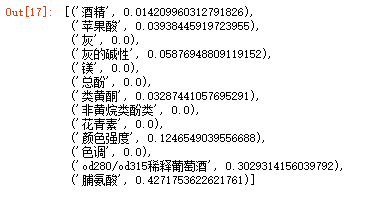``````clf = tree.DecisionTreeClassifier(criterion="entropy",random_state=30)
#可以使用"best"或者"random"。前者在特征的所有划分点中找出最优的划分点。后者是随机的在部分划分点中找局部最优的划分点。

clf = clf.fit(Xtrain, Ytrain)
score = clf.score(Xtest, Ytest) #返回预测的准确度
score``````

## 二、调参数  random_state & splitter

random_state用来设置分枝中的随机模式的参数，默认None，在高维度时随机性会表现更明显，低维度的数据
（比如鸢尾花数据集），随机性几乎不会显现。输入任意整数，会一直长出同一棵树，让模型稳定下来。
splitter也是用来控制决策树中的随机选项的，有两种输入值，输入”best"，决策树在分枝时虽然随机，但是还是会

``````clf = tree.DecisionTreeClassifier(criterion="entropy",random_state=30,splitter="random")
clf = clf.fit(Xtrain, Ytrain)
score = clf.score(Xtest, Ytest)
score
import graphviz
dot_data = tree.export_graphviz(clf,feature_names= feature_name,class_names=["琴酒","雪莉","贝尔摩德"],filled=True,rounded=True)
graph = graphviz.Source(dot_data)
graph``````

### 剪枝参数

``````#我们的树对训练集的拟合程度如何？
score_train = clf.score(Xtrain, Ytrain)
score_train``````

``````#带有剪枝参数
clf = tree.DecisionTreeClassifier(criterion="entropy"
,random_state=30
,splitter="random"
,max_depth=3
,min_samples_leaf=10
,min_samples_split=10
)
clf = clf.fit(Xtrain, Ytrain)
dot_data = tree.export_graphviz(clf
,feature_names= feature_name
,class_names=["琴酒","雪莉","贝尔摩德"]
,filled=True
,rounded=True
)
graph = graphviz.Source(dot_data)
graph
clf.score(Xtrain,Ytrain)
clf.score(Xtest,Ytest)``````

``````import matplotlib.pyplot as plt
test =[]
for i in range(10):
clf = tree.DecisionTreeClassifier(max_depth=i+1,criterion="entropy",random_state=30,splitter="random")
clf = clf.fit(Xtrain, Ytrain)
score = clf.score(Xtest, Ytest)
test.append(score)
plt.plot(range(1,11),test,color="red",label="max_depth")
plt.legend()
plt.show()``````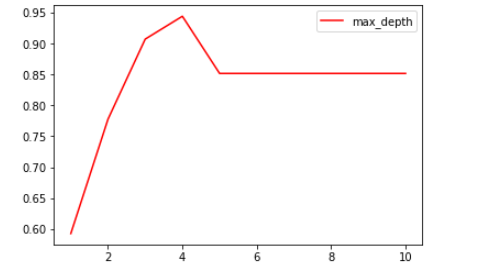### 目标权重参数

class_weight & min_weight_fraction_leaf

weight_fraction_leaf这个基于权重的剪枝参数来使用。另请注意，基于权重的剪枝参数（例如min_weight_
fraction_leaf）将比不知道样本权重的标准（比如min_samples_leaf）更少偏向主导类。如果样本是加权的，则使

### 重要属性和接口

sklearn中许多算法的接口都是相似的，比如说我们之前已经用到的fit和score，几乎对每个算法都可以使用。除了

``````#apply返回每个测试样本所在的叶子节点的索引
clf.apply(Xtest)``````
``````#predict返回每个测试样本的分类/回归结果
clf.predict(Xtest)``````

# 实例：分类树在合成数集上的表现

### 1、导入需要的库

``````import numpy as np
import matplotlib.pyplot as plt
from matplotlib.colors import ListedColormap
from sklearn.model_selection import train_test_split
from sklearn.preprocessing import StandardScaler
from sklearn.datasets import make_moons, make_circles, make_classification
from sklearn.tree import DecisionTreeClassifier``````

### 2. 生成三种数据集

``````#make_classification库生成随机的二分型数据
X, y = make_classification(n_samples=100, #生成100个样本
n_features=2,  #包含2个特征，即生成二维数据
n_redundant=0, #添加冗余特征0个
n_informative=2, #包含信息的特征是2个
random_state=1,  #随机模式1
n_clusters_per_class=1 #每个簇内包含的标签类别有1个
)
#在这里可以查看一下X和y，其中X是100行带有两个2特征的数据，y是二分类标签
#也可以画出散点图来观察一下X中特征的分布
#plt.scatter(X[:,0],X[:,1])
#从图上可以看出，生成的二分型数据的两个簇离彼此很远，这样不利于我们测试分类器的效果，因此我们使用np生成

#注意，这个过程只能够运行一次，因为多次运行之后X会变得非常稀疏，两个簇的数据会混合在一起，分类器的效应会

rng = np.random.RandomState(2) #生成一种随机模式
X += 2 * rng.uniform(size=X.shape) #加减0~1之间的随机数
linearly_separable = (X, y) #生成了新的X，依然可以画散点图来观察一下特征的分布
#plt.scatter(X[:,0],X[:,1])
#用make_moons创建月亮型数据，make_circles创建环形数据，并将三组数据打包起来放在列表datasets中
datasets = [make_moons(noise=0.3, random_state=0),
make_circles(noise=0.2, factor=0.5, random_state=1),
linearly_separable]``````

### 画出三种数据集和三棵决策树的分类效应图像

``````#创建画布，宽高比为6*9
figure = plt.figure(figsize=(6, 9))
#设置用来安排图像显示位置的全局变量i
i = 1
#开始迭代数据，对datasets中的数据进行for循环
for ds_index, ds in enumerate(datasets):

#对X中的数据进行标准化处理，然后分训练集和测试集
X, y = ds
X = StandardScaler().fit_transform(X)
X_train, X_test, y_train, y_test = train_test_split(X, y, test_size=.4,
random_state=42)

#找出数据集中两个特征的最大值和最小值，让最大值+0.5，最小值-0.5，创造一个比两个特征的区间本身更大

x1_min, x1_max = X[:, 0].min() - .5, X[:, 0].max() + .5
x2_min, x2_max = X[:, 1].min() - .5, X[:, 1].max() + .5

#用特征向量生成网格数据，网格数据，其实就相当于坐标轴上无数个点
#函数np.arange在给定的两个数之间返回均匀间隔的值，0.2为步长
#函数meshgrid用以生成网格数据，能够将两个一维数组生成两个二维矩阵。
#如果第一个数组是narray，维度是n，第二个参数是marray，维度是m。那么生成的第一个二维数组是以
narray为行，m行的矩阵，而第二个二维数组是以marray的转置为列，n列的矩阵
#生成的网格数据，是用来绘制决策边界的，因为绘制决策边界的函数contourf要求输入的两个特征都必须是二

array1,array2 = np.meshgrid(np.arange(x1_min, x1_max, 0.2),
np.arange(x2_min, x2_max, 0.2))
#接下来生成彩色画布
#用ListedColormap为画布创建颜色，#FF0000正红，#0000FF正蓝
cm = plt.cm.RdBu
cm_bright = ListedColormap(['#FF0000', '#0000FF'])

#在画布上加上一个子图，数据为len(datasets)行，2列，放在位置i上
ax = plt.subplot(len(datasets), 2, i)
#到这里为止，已经生成了0~1之间的坐标系3个了，接下来为我们的坐标系放上标题
#我们有三个坐标系，但我们只需要在第一个坐标系上有标题，因此设定if ds_index==0这个条件
if ds_index == 0:
ax.set_title("Input data")

#将数据集的分布放到我们的坐标系上
#先放训练集
ax.scatter(X_train[:, 0], X_train[:, 1], c=y_train,
cmap=cm_bright,edgecolors='k')
#放测试集
ax.scatter(X_test[:, 0], X_test[:, 1], c=y_test,
cmap=cm_bright, alpha=0.6,edgecolors='k')

#为图设置坐标轴的最大值和最小值，并设定没有坐标轴
ax.set_xlim(array1.min(), array1.max())
ax.set_ylim(array2.min(), array2.max())
ax.set_xticks(())
ax.set_yticks(())

#每次循环之后，改变i的取值让图每次位列不同的位置
i += 1

#至此为止，数据集本身的图像已经布置完毕，运行以上的代码，可以看见三个已经处理好的数据集

#############################从这里开始是决策树模型##########################

#迭代决策树，首先用subplot增加子图，subplot(行，列，索引)这样的结构，并使用索引i定义图的位置
#在这里，len(datasets)其实就是3，2是两列
#在函数最开始，我们定义了i=1，并且在上边建立数据集的图像的时候，已经让i+1,所以i在每次循环中的取值

ax = plt.subplot(len(datasets),2,i)

#决策树的建模过程：实例化 → fit训练 → score接口得到预测的准确率
clf = DecisionTreeClassifier(max_depth=5)
clf.fit(X_train, y_train)
score = clf.score(X_test, y_test)

#绘制决策边界，为此，我们将为网格中的每个点指定一种颜色[x1_min，x1_max] x [x2_min，x2_max]
#分类树的接口，predict_proba，返回每一个输入的数据点所对应的标签类概率
#类概率是数据点所在的叶节点中相同类的样本数量/叶节点中的样本总数量
#由于决策树在训练的时候导入的训练集X_train里面包含两个特征，所以我们在计算类概率的时候，也必须导入

#ravel()能够将一个多维数组转换成一维数组
#np.c_是能够将两个数组组合起来的函数
#在这里，我们先将两个网格数据降维降维成一维数组，再将两个数组链接变成含有两个特征的数据，再带入决策

Z = clf.predict_proba(np.c_[array1.ravel(),array2.ravel()])[:, 1]

#np.c_[np.array([1,2,3]), np.array([4,5,6])]

#将返回的类概率作为数据，放到contourf里面绘制去绘制轮廓
Z = Z.reshape(array1.shape)
ax.contourf(array1, array2, Z, cmap=cm, alpha=.8)

#将数据集的分布放到我们的坐标系上
# 将训练集放到图中去
ax.scatter(X_train[:, 0], X_train[:, 1], c=y_train, cmap=cm_bright,
edgecolors='k')
# 将测试集放到图中去
ax.scatter(X_test[:, 0], X_test[:, 1], c=y_test, cmap=cm_bright,
edgecolors='k', alpha=0.6)

#为图设置坐标轴的最大值和最小值
ax.set_xlim(array1.min(), array1.max())
ax.set_ylim(array2.min(), array2.max())
#设定坐标轴不显示标尺也不显示数字
ax.set_xticks(())
ax.set_yticks(())

#我们有三个坐标系，但我们只需要在第一个坐标系上有标题，因此设定if ds_index==0这个条件
if ds_index == 0:
ax.set_title("Decision Tree")

#写在右下角的数字
ax.text(array1.max() - .3, array2.min() + .3, ('{:.1f}%'.format(score*100)),
size=15, horizontalalignment='right')

#让i继续加一
i += 1
plt.tight_layout()
plt.show()``````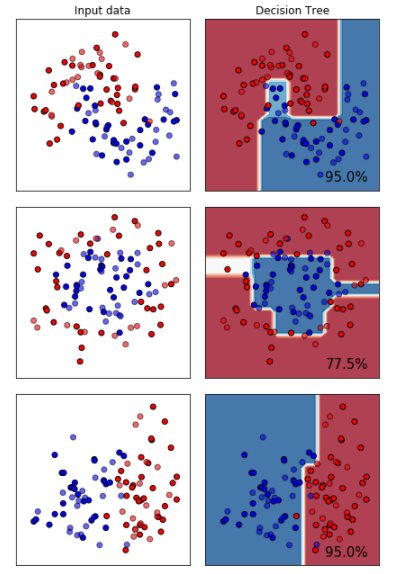### ES6_等待戈多的莱昂纳多的博客-程序员资料

var 1. 可以重复声明 2. 无法限制修改 3. 没有块级作用域let 不能重复声明 变量-可以修改，块级作用域const 不能重复声明，常量-不能修改，块级作用域

### CountDownLatch 的 .await() 的线程阻塞 和countDown() 计时唤醒_清风羽的博客-程序员资料_countdownlatch.await

CountDownLatch是一种java.util.concurrent包下一个同步工具类，它允许一个或多个线程等待直到在其他线程中一组操作执行完成。CountDownLatch的用法非常简单，下面的例子也是我在网上看到的，十分贴切，这里就贴出来public class Test { public static void main(String[] args) { ...

### 【操作系统】Cache与主存之间的全相联映射，直接映射和组相联映射的区别_小辣抓的博客-程序员资料_组相联映射

Cache与主存之间的全相联映射，直接映射和组相联映射的区别1.高速缓冲存储器的功能、结构与工作原理　　高速缓冲存储器是存在于主存与CPU之间的一级存储器， 由静态存储芯片(SRAM)组成，容量比较小但速度比主存高得多，接近于CPU的速度。 Cache的功能是用来存放那些近期需要运行的指令与数据。目的是提高CPU对存储器的访问速度。为此需要解决2个技术问题：一是主存地址与缓存地址的映象及转换； 二是

### Java字符串处理之返回指定字符串下标（indexOf）_Claroja的博客-程序员资料_做一个字符串搜索函数,从一字符串里面搜索字符,返回字符下标的位置,用数组实现

Java中使用indexOf或lastIndexOf方法来获得指定字符在字符串里第一次出现的下标。 　　1.indexOf 　　public int indexOf(String str, int fromIndex)查找String str在字符串里的第一次出现的下标，从int fromIndex开始，返回值是整型。String str = "wanag";str.indexOf("a"

### mac下用brew安装的mysql配置文件路径_yahaha我去的博客-程序员资料

【MySQL】Mac 上使用 brew 安装的 mysql 配置文件位置在mac 上使用 brew 安装 mysql 后，发现 /etc/my.cnf 并不存在。于是使用命令查找 MySQL 的配置启动文件列表：\$ mysqld --help --verbose | less...Default options are read from the following files...